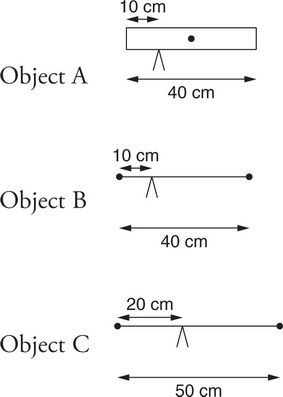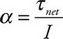# AP Physics 1 Question 148: Answer and Explanation

### Test Information

Question: 148

3.Three equal-mass objects (A, B, and C) are each initially at rest horizontally on a pivot, as shown in the figure. Object A is a 40 cm long, uniform rod, pivoted 10 cm from its left edge. Object B consists of two heavy blocks connected by a very light rod. It is also 40 cm long and pivoted 10 cm from its left edge. Object C consists of two heavy blocks connected by a very light rod that is 50 cm long and pivoted 20 cm from its left edge. Which of the following correctly ranks the objects’ angular acceleration about the pivot point when they are released?

• A. A = B > C
• B. A > B = C
• C. A < B < C
• D. A > B > C

D-Angular acceleration is net torque divided by rotational inertia,. Imagine each object has total mass of 2 kg. Begin by comparing objects A and B: To find the net torque on object A, assume the entire 20 N weight is concentrated at the dot representing the rod's center of mass. That's located 10 cm from the pivot, giving a net torque of 200 N·cm. For object B, consider the torques provided by each block separately. The right block provides a torque of (10 N)(30 cm) = 300 N·cm clockwise; the left block provides a torque of (10 N)(10 cm) = 100 N·cm counterclockwise. That makes the net torque 200 N·cm, the same as for object A. But object B has more rotational inertia, since its 2 kg of mass are concentrated farther away from the pivot than object A's mass. So the denominator of the angular acceleration equation is bigger for object B with the same numerator, which means A > B.